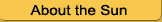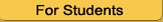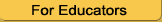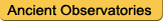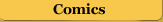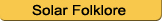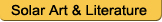Estimating the Sun's Rotation Period

### Estimating the Sun's Rotation Rate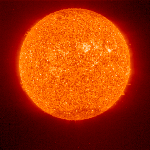How can you use your sunspot data to figure out how long it takes for the Sun to spin around once? To estimate the Sun's rotation rate, let's assume that the Sun is a flat disk, just like it appears on your copies or sketches.

You can use a calculator for this exercise.On your data sketches, pick a sunspot group which travels a long distance across the Sun's disk. A good choice would be a sunspot which starts out closest to the left limb (edge) of the Sun. Let's call this spot "George". You are going to figure out how long it took George to move across the Sun.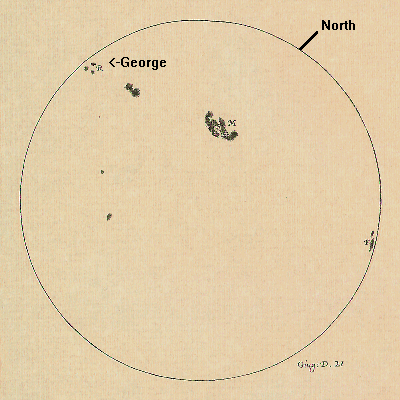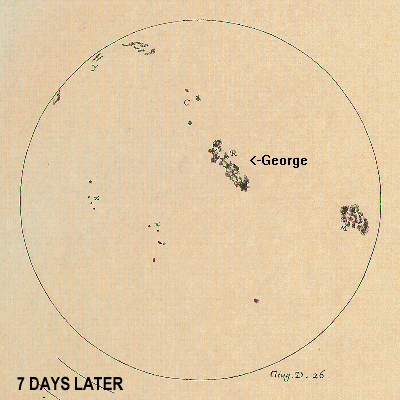Find your picture with George closest to the left limb of the Sun. With a (metric) ruler, measure how far away from the left edge of the Sun's disk George is.Now, find the picture with George closest to the right limb of the Sun and measure it's distance. Make sure you again measure the distance starting from the left limb.Now, measure the distance across the entire disk of the Sun (ignoring George and any of his friends). You will need to multiply this by 2 to include the back side of the Sun.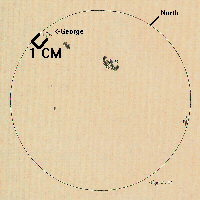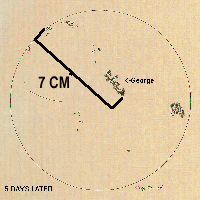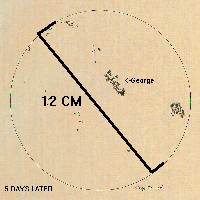Look again at your data sheets and find out what time your first sketch of George was taken. Find the time for your last sketch of George. How long did it take for George to travel from the first place to the last? (Subtract the last time from the first. In our example, it was 7 days.Now, how far around the Sun did George go? In our example, George went 6 cm (7 cm - 1 cm) and the Sun was 24 cm around. So, in this example, it took George 7 days to get 1/4 of the way around the Sun, which means that George would need 4*7 = 28 days to go all the way around (assuming he could last that long).If your numbers are more complicated than George's, then you can use your calulator to figure it out:

Sun's rotation time = George's-time * (Sun's-distance / George's-distance)
28 days = 7 days * (24 cm/6 cm)

If you picked a different spot or group, do you think your answer for
the Sun's rotation rate would be the same?
Try to find out by doing the calculation for groups at higher or lower
latitudes (that is, groups that are closer or farther from the Sun's
poles).

You have just estimated the solar rotation rate. Your data will be more accurate if you use the Angular Velocity exercize.

```
```